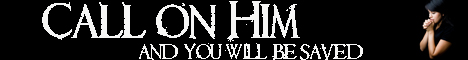Note:  Do not rely on this information. It is very old.

# Epicycloid

Epicycloid, in Geometry, is the curve traced out by a point on the circumference of a circle that rolls round another. If the fixed circle becomes greater and greater, so as to approximate to a straight line, the epicycloid approximates to the cycloid (q.v.). If the rolling circle move on the concave side of the fixed curve it generates the hypocycloid. If the two are of equal magnitude, the point on. the, rolling circle just describes one closed curve in one revolution; this curve is the cardioid. Of hypocycloids may be mentioned the four-cusp, formed when the rolling circle is one quarter the diameter of the fixed circle. This curve is the envelope of a line of fixed length moving so that its extremities are kept in two lines at right angles to each other. If the inner circle is one half the diameter of the outer, the special hypocycloid obtained is the straight line. Such curves were once important in astronomy. [Epicycle.]